Construction of Decision Tree for "Play Tennis?"

Values(Wind) = {weak, strong}

S = [9+, 5-]

Sweak = [6+, 2-]= .811

Sstrong = [3+, 3-]= 1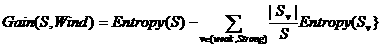Values(Outlook) = (rain, overcast, sunny)

Srain = [3+, 2-]=.971

Sovercast = [2+, 2-]=0

Ssunny = [2+, 3-]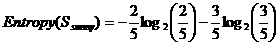=.971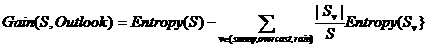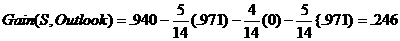Gain(S, Temperature) = .029

Outlook has the highest Information Gain and thus classifies the root node.

[D1, D2, D8, D9, D11]

[2+, 3-]

S = .971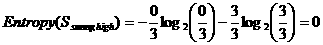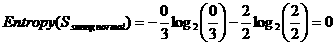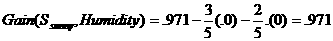Gain(Ssunny, Temperature) = .570

Gain(Ssunny, Wind) = .570